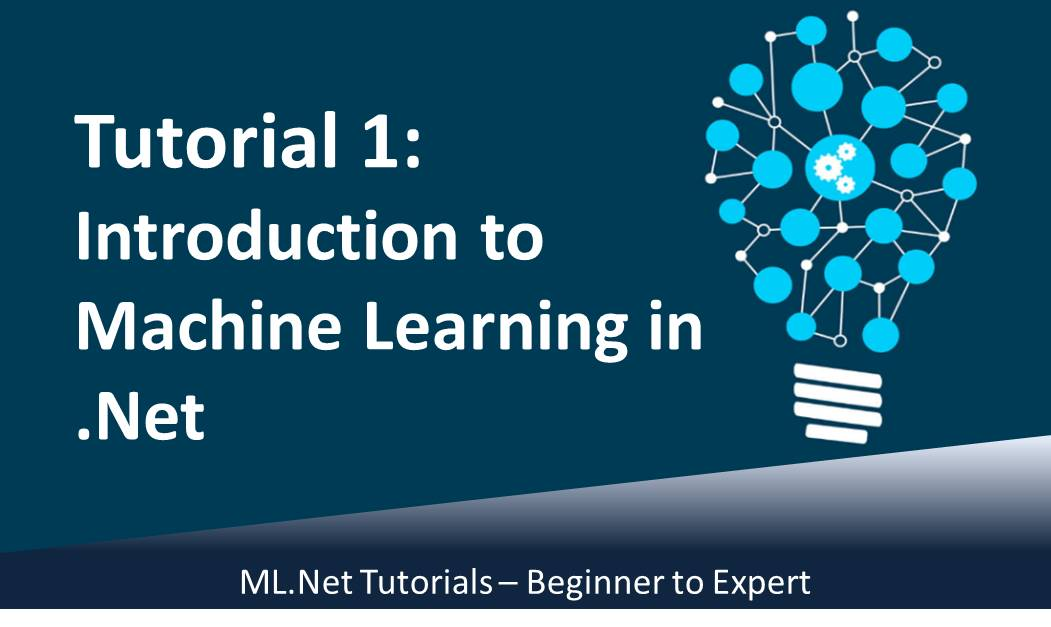# ML.Net Tutorial 1: Introduction to Machine Learning in .Net (Beginner to Expert)My name is Kindson The Genius and today, I would introduce you to Machine Learning using .Net. Yes, .net C# programmer can now develop Machine Learning models using the .net framework in visual studio.

In this series of tutorials, I would show you exactly how to do this. We would cover the following:

You will also be happy to know that Machine Learning is a very clear and easy to follow topic very much unlike the sound of the name.

#### 1. What is Machine Learning?

Maching Learning is simply a situation where the computer examines a dataset and then use the information to make prediction.
This is the same thing we do everyday, but this time we want the compute to do it simply because it would be faster.

Take an example
Take a look at the table below. The color of the fruit and the weight is provided, and then the last column contains the type of fruit.

In the last record, what do you think would be the type of fruit? You guess right! It would be Grape. The reason follows from the  previous records.
Since grapes in the previous records have a weigh of about 8 to 10 and has a color of green, therefore this must be grape.

Now the color and the weight are called features. The Fruit is called the Class in machine language terms. In this case, there are two classes: Apple and Grape and also two features: Color and Weight.

This is what machine learning is all about: examine the features and determine the class.

This is a typical example of Classification, a branch of machine learning. The other branches are Regression and Clustering.

#### 2. Machine Learning in .Net

Microsoft have provided a package called ML.net that can be added to Visual Studio and used to solve Machine Learning Problems.

To create machine learning problem, there are four steps to follow:

Step 1: Create a new Project
Step 2: Add your data into the project
Step 3: Train the model (the system examines your data to understand it)
Step 4: Make prediction on new data

This are the basic steps we would follow in Tutorial 2 to create a model and use it to make prediction.

#### 3. How to Set up ML.Net

Before you continue to Tutorial 2, do the following:

• Create a new Solution in Visual Studio
• Add ML.Net Package to your Solution from the Nugget Manager